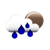### Percentage Error Calculator

 Example: What is the percentage error between: 200 (approximate value) and 180 (actual value) ? Anwser: (200 - 180) ÷ 180 × 100 = 11.11% What is the percentage error between: Approximate Value: Actual Value: ? Answer: %

The Percentage Error Calculator tool enables you to calculate the percentage error percentage error between an approximate value (or experimental measured value) and a theoretical actual value.

#### The Percent Error Formula

You can use the percent error equation to find the percentage error of two value:

Percentage Error
=
Approximate Value - Actual Value / Actual Value
× 100

Example: We project 100 people will attend the seminar. However, only 80 people has attended the seminar. What is the percentage error ?

Percentage Error
=
100 - 80 / 80
× 100

Anwser: 25%

To know how to calculate percent error between two numbers? Please use our percentage error calculator.

#### References

More references for Percentage Error Definition

#### Local Weather Status

Ashburn, United States
9th December, 2019 Monday
Light Rain6.67 - 10
Humidity: 93 %
Wind: 6.2 km/h